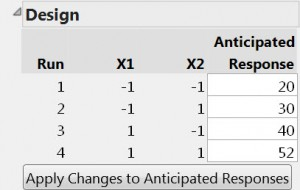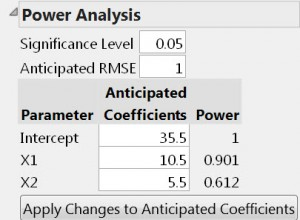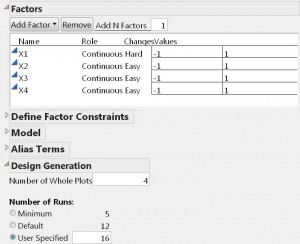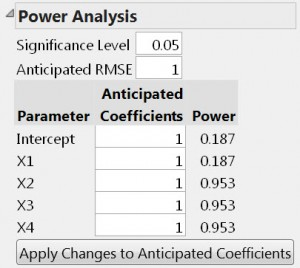Our World Statistics Day conversations have been a great reminder of how much statistics can inform our lives. Do you have an example of how statistics has made a difference in your life? Share your story with the Community!
Choose Language Hide Translation Bar
JMP 11: Custom Design power calculations – Part II

Last week, I wrote about the Custom Design user interface for power calculations that is new for JMP 11. My goal for this week’s blog post is to explain how to interpret the new power analysis for the two-level screening designs including screening with hard-to-change factors.

What is so different about screening designs?

The fundamental conceptual framework for power is general and includes screening designs. Many textbooks, however, do not explain the analysis of screening experiments using regression. However, the Fit Model platform provides a natural way to analyze such experiments in JMP. Since the Fit Model platform uses the method of least squares regression for its parameter estimation, users of screening experiments with traditional training in design of experiments (DOE) need an explanation that clarifies the terminology.

So what are these terms that need explanation?

The analysis of screening experiments is often presented in terms of the effects of the factors – namely main effects and interaction effects. The analysis of regression models yields parameter estimates. Though the two different analytical approaches give the same predicted responses for orthogonal designs, factor effects are not the same as parameter estimates. So, it is important to define our terms.

According to Montgomery (2013), the “effect of a factor is defined to be the change in the response produced by a change in the level of the factor.” Figure 1 shows the data for a specific example (Montgomery, pages 184–186) showing the factor effects and the regression model.The main effect of X1 is the difference between the average response at the high level of X1 [(52+40)/2 = 46] and the average response at the low level of X1 [(20+30)/2 = 25]. The difference in the averages or the main effect is 46 – 25 = 21. A similar calculation for factor X2 yields a main effect of 11. We say that the main effect of changing X1 from low to high is that the response increases by 21 units. The main effect of changing X2 from low to high is an 11-unit increase in the response.

As Montgomery points out, another way of looking at this data is the regression model representation: y = β0 + β1x1  + β2x2 + ε

The estimates of β1 and β2 are one-half the value of their corresponding main effects. This is because the main effect is the difference in the response going from a scaled X-value of -1 to a scaled X-value of +1. That is a change of two units. The coefficients  β1 and β2, however, are interpreted as slopes (i.e. the change in the response due to a one unit change in the factor). For the data in Figure 1, the equation for the predicted responses is: y = 35.5 + 10.5x1  + 5.5x2. JMP uses the regression model representation, as you can see by examining Figure 2.Note that the power for detecting a regression coefficient as large as 10.5 is 0.901. That is the same as the power for detecting a main effect of 21. Some implementations of power calculations define the power in terms of effects rather than regression parameters (or coefficients). To match JMP’s results with these implementations, you would need to multiply the Anticipated Coefficients values by two.

Why doesn’t JMP use factor effects instead of regression coefficients?

First, it is somewhat unclear how to define a factor’s effect (signal) when the factor has more than two levels. Once you define a coding convention for multilevel factors, the regression coefficients are uniquely defined. This was covered in my previous blog post.

Second, calculating effects by the difference in the averages of responses at the high and low levels of the factors works well for designs that are orthogonal. While textbook screening designs are orthogonal, data that arise in practice are often nonorthogonal due to the loss of a run, partial replication or a number of runs (discounting center runs) that is not a multiple of four. When, the data are nonorthogonal, calculating effects as a difference in averages does not provide the best estimates. Fitting a regression model by least squares is preferable. The regression approach is more general.

What about power calculations for split-plot experiments?

When certain factors are hard to change from one run to the next, it is desirable to run experiments where such factors stay constant for an entire group of runs. Experiments with this structure are called split-plot experiments.

In one sense, a split-plot experiment is like two experiments in one. The smaller experiment involves the factor(s) that are hard to change. The number of groups of runs where the hard to change factors remain constant is the sample size for that experiment. The larger experiment consists of all the runs.

How about an example for clarification?

Suppose you have one hard-to-change factor and three easy-to-change factors, and you want to run your experiment in four groups of four runs where the hard-to-change factor stays constant in each group. Figure 3 shows the specification of this example using the Custom Design tool.The power analysis shown in Figure 4 illustrates the fact that the power for a hard-to-change factor is generally substantially lower than the power for the other factors.How come the coefficient for X1 has such low power when it is the same size as the other coefficients?

There are two reasons for the low power for X1. First, there are only four groups of runs where X1 changes. In essence then, the experiment in the hard-to-change factor has only four runs. The Intercept and the coefficient for X1 both count as model degrees of freedom for this four-run design so there are only two degrees of freedom for error. By comparison, the other coefficients have 11 error degrees of freedom (16 runs - 5 unknowns = 11 degrees of freedom). Second, the variance of the X1 effect has two components. One component, shared by the other coefficients, is the usual run-to-run variance. The other component is the variance due to the random kick to the process coming from changing the hard-to-change factor. The variance of the X1 coefficient relative to σ2 is 5/16, which is five times larger than the variance of the other coefficients.

Note that the variance of the X1 effect depends on the ratio of the two variances above. By default, that ratio is 1. Also note, that the Custom Design tool now keeps track of the error degrees of freedom for every effect. So, it is no longer necessary to supply an edit box for this quantity as it was in JMP 10.

Where did the Signal to Noise Ratio go?

JMP 10 had a single number called the Signal to Noise Ratio. In JMP 11, this one number has been replaced by two or more numbers. The signal part of the ratio comes from the Anticipated Coefficients for each effect. The noise part of the ratio is the Anticipated RMSE. The fitted RMSE is the number JMP uses to estimate σ.

Any final words?

The work on power calculations is not finished with the implementation in JMP 11. There are two enhancements I see coming in future releases.

First, some experiments do not have continuous responses with random normal variability. Another possible response for a run is one of two alternatives – success or failure. In such cases, the power of an effect of a given size is necessarily lower because a 0/1 response contains less information than a continuous response. Providing power calculations for these binary responses would be desirable.

Second, the current implementation requires you to generate multiple designs with differing numbers of runs to ascertain the relationship between power and sample size for a particular set of requirements. Providing an automated mechanism for generating power versus sample size curves would be useful, too.

Article Labels

There are no labels assigned to this post.

Article Tags
Visitor

Mike Clayton wrote:

You indicated future patch or later release that would provide "an automated mechanism for generating power versus sample size curves would be useful" and that sounds great for engineers like us who are not statisticians. And thanks for the carefully worded and useful blog tutorials. Hoping to test out JMP 11 soon.

Visitor

Doyle Boese wrote:

How is the power calculated for the effect of a categorical factor with greater than 2 levels in an unbalanced design? I have found documentation supporting calculating the individual contrasts but not the "effect."Staff